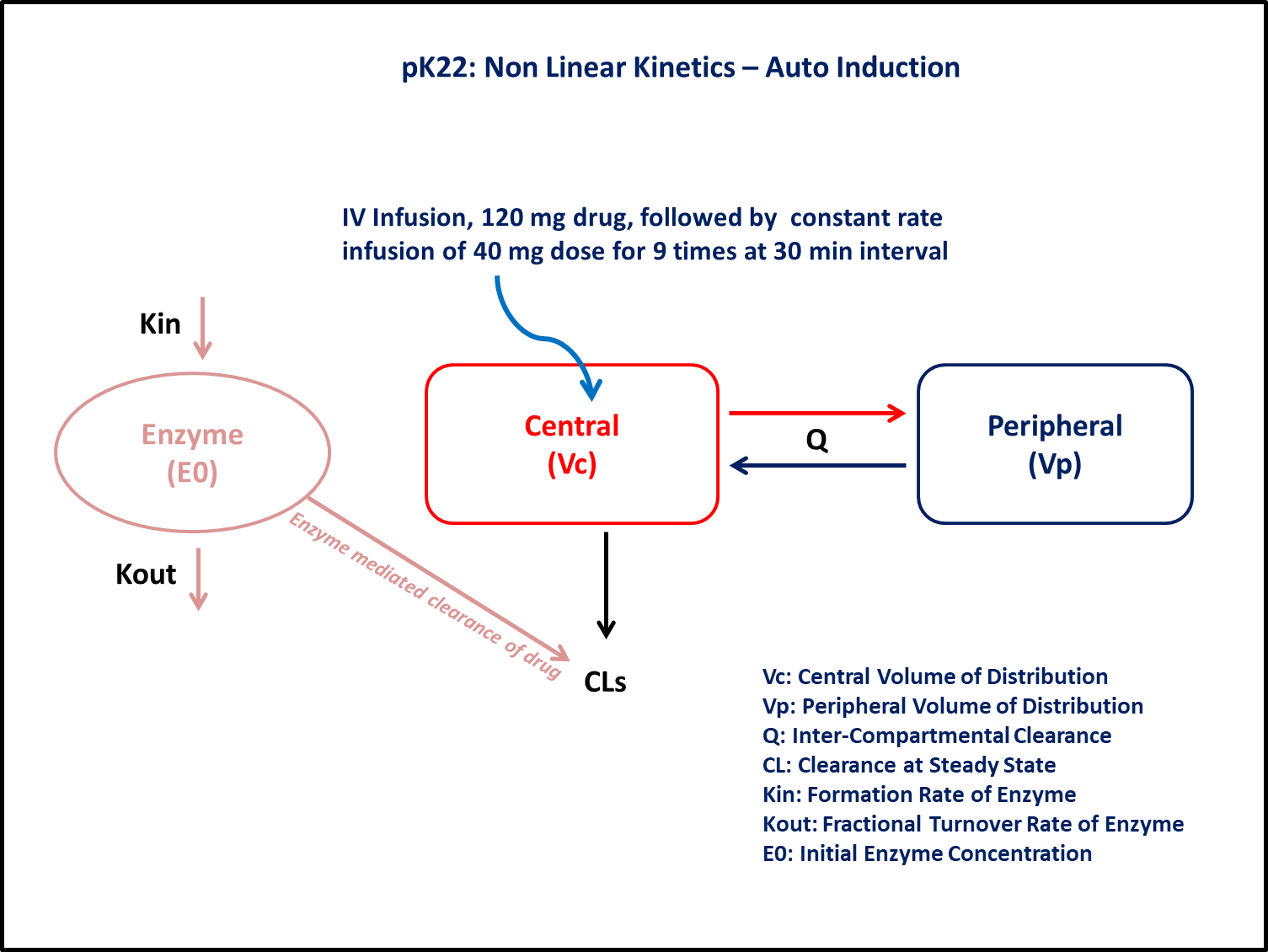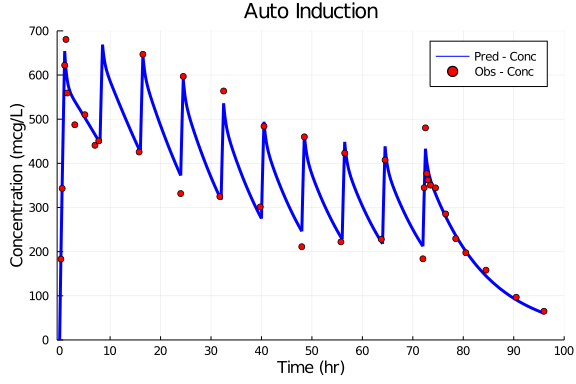# Exercise PK22 - Non Linear Kinetics - Auto Induction

### Background

• Structural model - Two compartment Model for drug and one compartment model for enzyme

• Route of administration - IV Infusion

• Dosage Regimen - 120 mg of IV infusion with constant rate of 1 hr followed by 9 more doses of 40 mg for 30 minutes duration at the interval of 8 hrs

• Number of Subjects - 1### Learning Outcome

This model gives understanding of drug pharmacokinetics and enzyme autoinduction simultaneously after repeated IV infusion.

### Objectives

In this tutorial, you will learn how to build a two compartment model for a drug and one compartment model for an enzyme and simulate the model.

### Libraries

call the "necessary" libraries to get started.

using Pumas
using Plots
using CSV
using StatsPlots
using Random


### Model

In this two compartment model, we administer repeated dose of IV infusion to a single subject.

pk_22            = @model  begin
@param begin
tvcls       ∈ RealDomain(lower=0)
tvvc        ∈ RealDomain(lower=0)
tvvp        ∈ RealDomain(lower=0)
tvq         ∈ RealDomain(lower=0)
tvkin       ∈ RealDomain(lower=0)
tvkout      ∈ RealDomain(lower=0)
tvE0        ∈ RealDomain(lower=0)
Ω           ∈ PDiagDomain(7)
σ²_prop     ∈ RealDomain(lower=0)
end

@random begin
η           ~ MvNormal(Ω)
end

@pre begin
Cls         = tvcls * exp(η)
Vc          = tvvc * exp(η)
Vp          = tvvp * exp(η)
Q           = tvq * exp(η)
Kin         = tvkin * exp(η)
Kout        = tvkout * exp(η)
E0          = tvE0 * exp(η)
end

@init begin
Enzyme      = (Kin/Kout)+ E0
end

@dynamics begin
Central'    = -(Cls/Vc) * Central * Enzyme + (Q/Vp) * Peripheral - (Q/Vc) * Central
Peripheral' =  (Q/Vc) * Central - (Q/Vp) * Peripheral
Enzyme'     =  Kin * (E0+Central/Vc) -  Kout * Enzyme
end

@derived begin
cp          = @. Central/Vc
dv          ~ @. Normal(cp, sqrt(cp^2*σ²_prop))
end
end

PumasModel
Parameters: tvcls, tvvc, tvvp, tvq, tvkin, tvkout, tvE0, Ω, σ²_prop
Random effects: η
Covariates:
Dynamical variables: Central, Peripheral, Enzyme
Derived: cp, dv
Observed: cp, dv


### Parameters

Hereby Parameters are provided for the simulation. tv represents the typical value for parameters.

• tvcls - Typical Value Clearance at Steady State (L/hr)

• tvvc - Typical Value Central Volume of Distribution (L)

• tvvp - Typical Value Peripheral Volume of Distribution (L)

• tvq - Typical Value Distribution Clearance (L/hr)

• tvkin - Input Rate Constant for Enzyme (hr⁻¹)

• tvkout - Output Rate Constant for Enzyme (hr⁻¹)

• tvE0 - Initial Enzyme Concentration

• Ω - Between Subject Variability

• σ - Residual errors

param =  (tvcls    = 0.04,
tvvc     = 150.453,
tvvp     = 54.0607,
tvq      = 97.8034,
tvkin    = 0.0238896,
tvkout   = 0.0238896,
tvE0     = 132.864,
Ω        = Diagonal([0.0,0.0,0.0,0.0,0.0,0.0,0.0]),
σ²_prop  = 0.005)

(tvcls = 0.04, tvvc = 150.453, tvvp = 54.0607, tvq = 97.8034, tvkin = 0.023
8896, tvkout = 0.0238896, tvE0 = 132.864, Ω = [0.0 0.0 … 0.0 0.0; 0.0 0.0 …
0.0 0.0; … ; 0.0 0.0 … 0.0 0.0; 0.0 0.0 … 0.0 0.0], σ²_prop = 0.005)


### Dosage Regimen

Dosage Regimen - 120 mg of IV infusion with constant rate of 1 hr followed by 9 more doses of 40 mg for 30 minutes duration at the interval of 8 hrs

ev1  = DosageRegimen([120000,40000], time=[0,8], duration=[1,0.5], cmt=[1,1], addl=[0,8], ii=[0,8])
sub1 = Subject(id=1, events=ev1)

Subject
ID: 1
Events: 20


### Simulation

Lets simulate for plasma concentration with the specific observation time points after IV.

Random.seed!(123)
sim_sub1 = simobs(pk_22,sub1,param,obstimes=0.00:0.01:96)
df1      = DataFrame(sim_sub1)


### DataFrame & Plots

Use the DataFrame for Plotting where

• cp - Predicted concentration

• dv - Observed concentration

df1_dv = filter(x -> x.time in [0.25,0.5,1,1.25,1.5,3,5,7,7.75,8.5,15.75,16.5,23.99,
24.5,31.75,32.5,39.75,40.5,47.99,48.5,55.75,56.5,63.75,64.5,72,72.25,72.5,72.75,
73,73.5,74.5,76.5,78.5,80.5,84.5,90.5,96], df1)

@df df1 plot(:time, :cp,
label="Pred - Conc",title="Auto Induction", linestyle=[:solid],  linewidth=3,
xlabel="Time (hr)", ylabel="Concentration (mcg/L)", color=[:blue],
xlims=(-0.5,100), xticks=[0,10,20,30,40,50,60,70,80,90,100],
yticks=[0,100,200,300,400,500,600,700], ylims=(0,700))
@df df1_dv scatter!(:time, :dv, label="Obs - Conc", color=[:red])